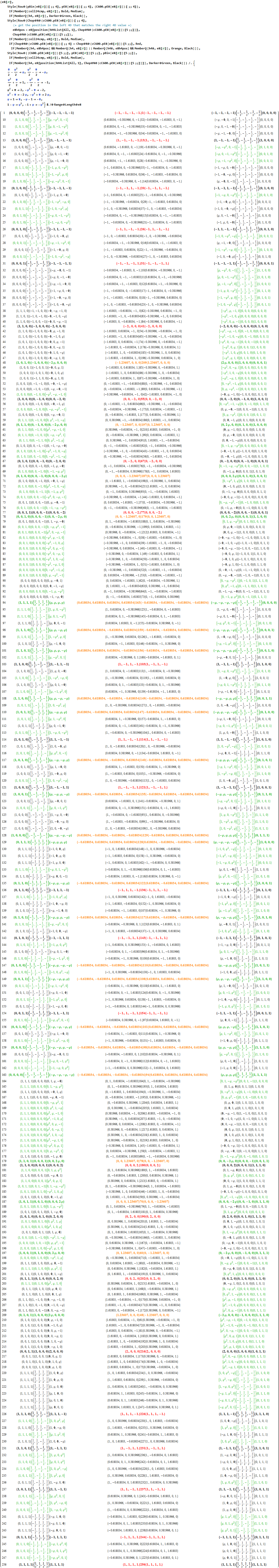# Metatron’s Cube

I was recently asked to create a 3D laser etched crystal of Metatron’s Cube.

The 13 Metatron rings are (or at least can be) taken from the 16 vertices of the tesseract (a 4D geometric figure called an 8-cell) which can be visualized by projecting 4D into 3D as two concentric 3D cubes.

Three of the 16 rings are hidden behind the ring in the front center when projecting the two concentric 3D cubes to 2D (or looking at it from just the correct angle on a corner and ignoring the perspective error introduced by parallax).

There are many more lines in the Metatron cube than from the typical 32 edges of the 8-cell tesseract connecting the vertices. These come from what is called the “complete graph” of 120 edges from the 16 vertices of the 8-cell. The Platonic solids are all contained (or related by duality) within the 3D projection of this complete graph of the 8-cell with large vertex spheres.

Slightly off angle (notice the 3 hidden spheres are now visible):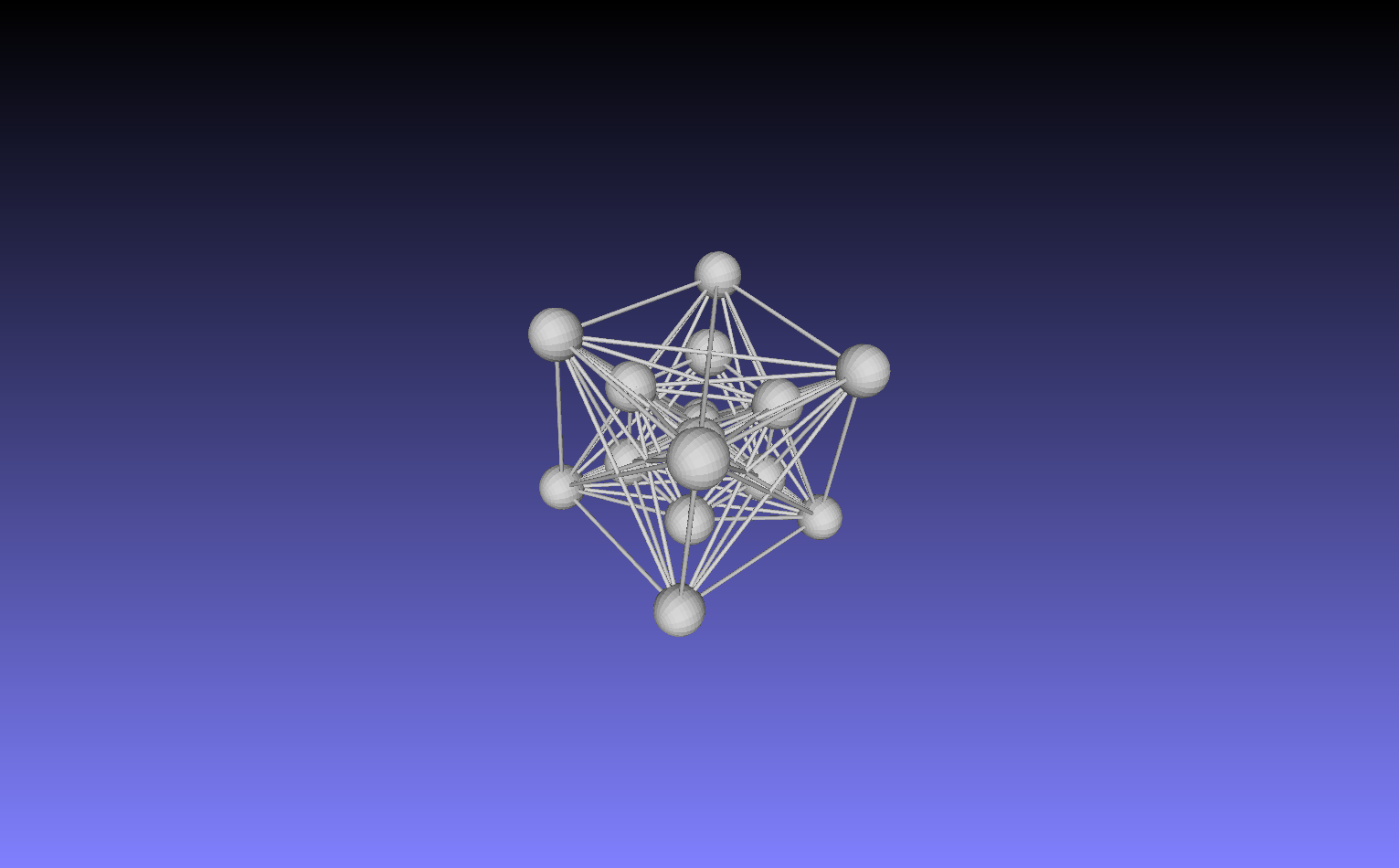# E8 (2_41, 4_21, and 1_42) projected to various Coxeter planes

This is a shot of the relevant Mathematica code snippets that produce the images:E8 4_21 (with projection basis, edges, vertex overlap colors & counts) (click here for very large SVG):E8 2_41 (with projection basis, vertex overlap colors & counts) (click here for very large SVG):

# A new ‘more Natural’ ToE model with Covariant Emergent Gravity as a solution to the dark sector

Please take a look at my latest paper based on my original work circa 1997-2007.
JGM/010-MOND-CEG-TOE [pdf, cite]
Title: A new ‘more Natural’ ToE model with Covariant Emergent Gravity as a solution to the dark sector
Authors: J Gregory Moxness
Comments: Apr 4 2018, 4 pages.
Subjects: General Relativity and Quantum Cosmology (gr-qc); High Energy Physics – Theory (hep-th)

For citations, remove the LaTex href tag structure if you don’t use the hyperref package.

# Tired of suffering the fools of Wikipedia Nazis

They really are a bunch of holier than thou bureaucrats with limited perspectives.  T. Ruin (sp) and JBL (like the speakers) and Dmcq – a cabal like no other.

# Perspective Enhanced HyperCube Projections to 3D

These objects are projected using an {x,y,z} orthonormal basis. For n={5,6,7} I add a small delta (.2) to {x,y,z} basis vectors in columns {n-2,n-1,n} in order to keep vertices from overlapping. There is an enhanced perspective applied to vertex location as well.

# 6 Demicubes Projected via H4 Folding Matrix

Taking the 32 vertex 6 Demicube with an even number of -1 elements and projecting with 3 of the 4 rows of the H4 folding matrix gives an dodecahedron hull with 12 vertices and 30 edges on the hull out of 240 edges of 6D length Sqrt).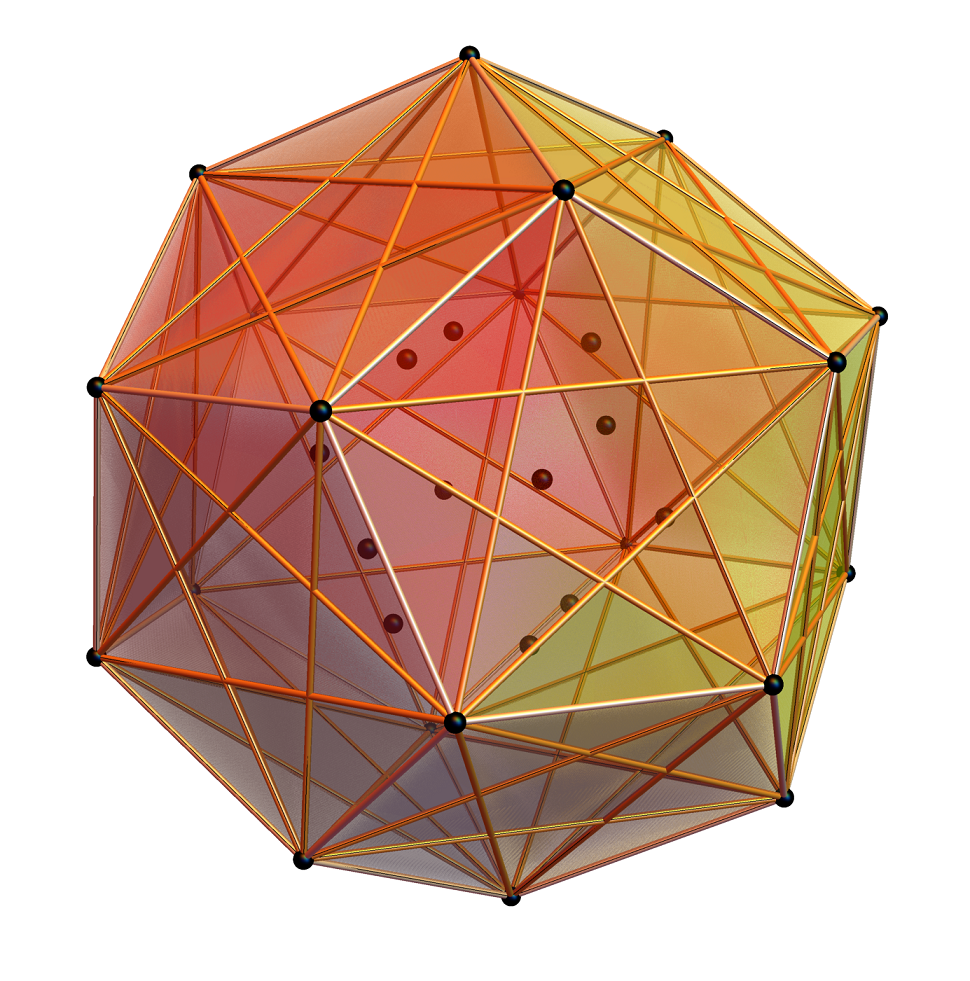BTW – if you find this information useful, or provide any portion of it to others, PLEASE make sure you cite this post. If you feel a blog post citation would not be an acceptable form for academic research papers, I would be glad to clean it up and put it into LaTex format in order to provide it to arXiv (with your academic sponsorship) or Vixra. Just send me a note at:  jgmoxness@theoryofeverthing.org.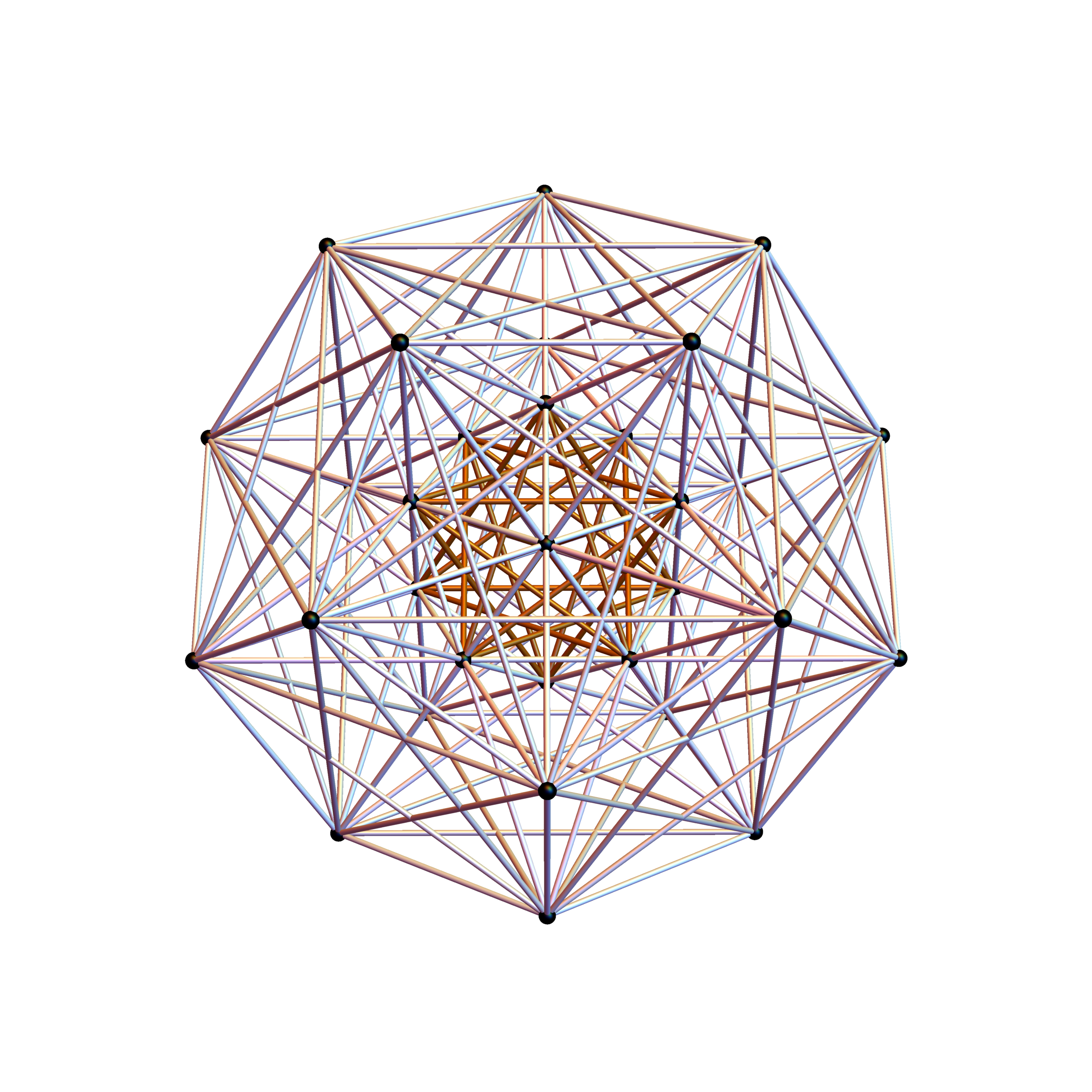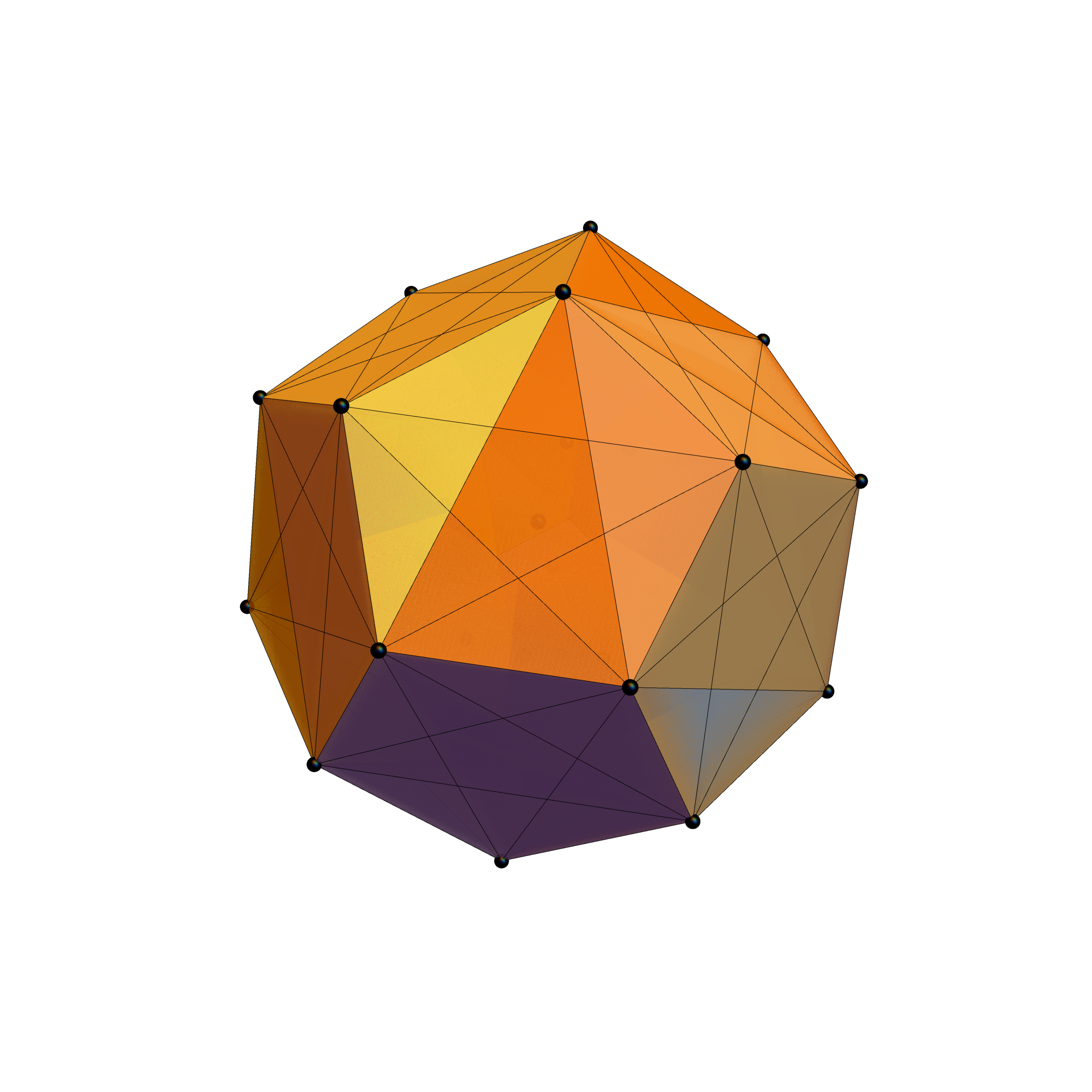While taking the 32 vertex 6 Demicube with odd number of -1 elements and projecting with 3 of the 4 rows of the H4 folding matrix gives the icosahedron with 20 vertices and 30 edges on the hull out of 240 edges of 6D length Sqrt).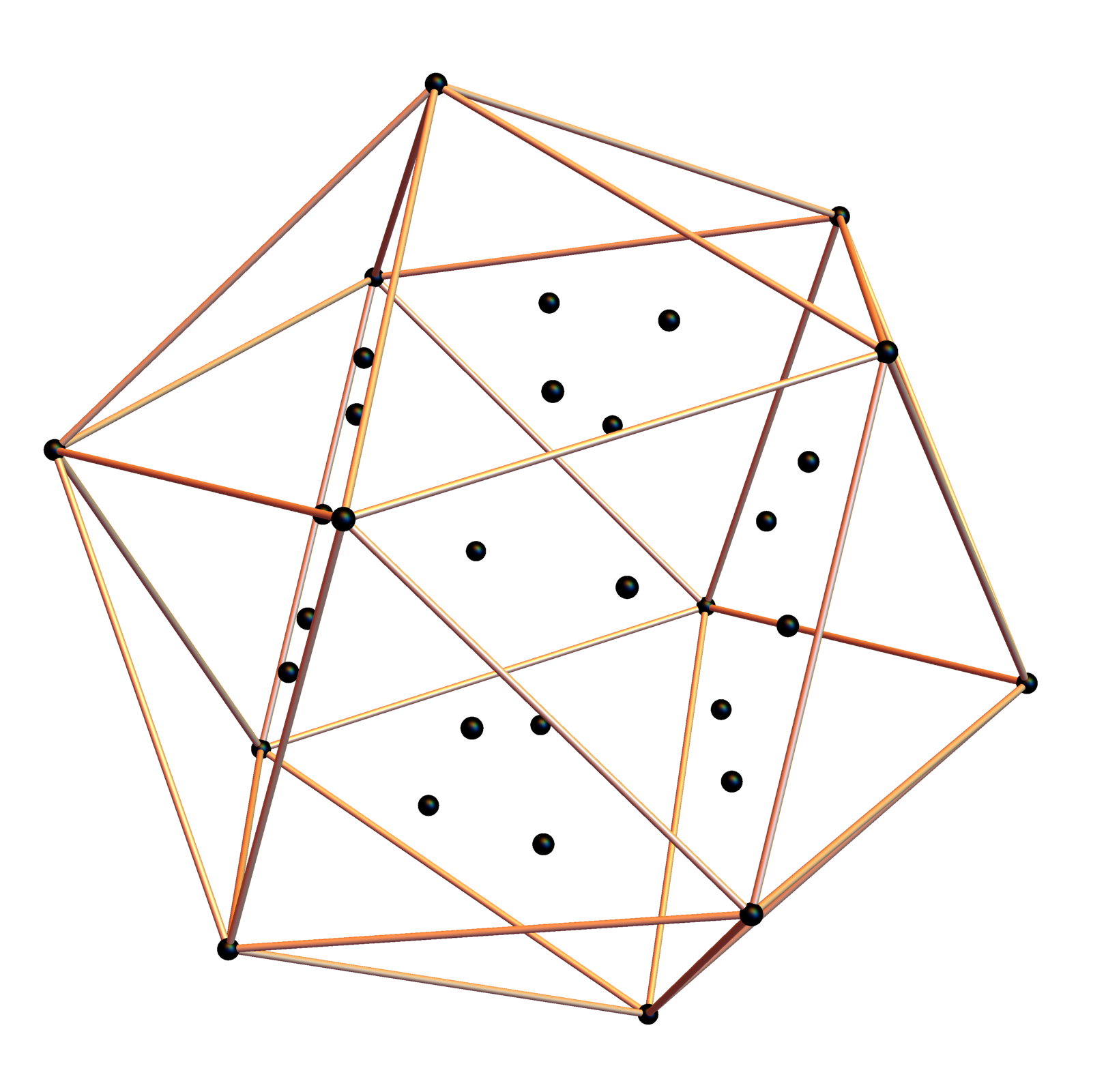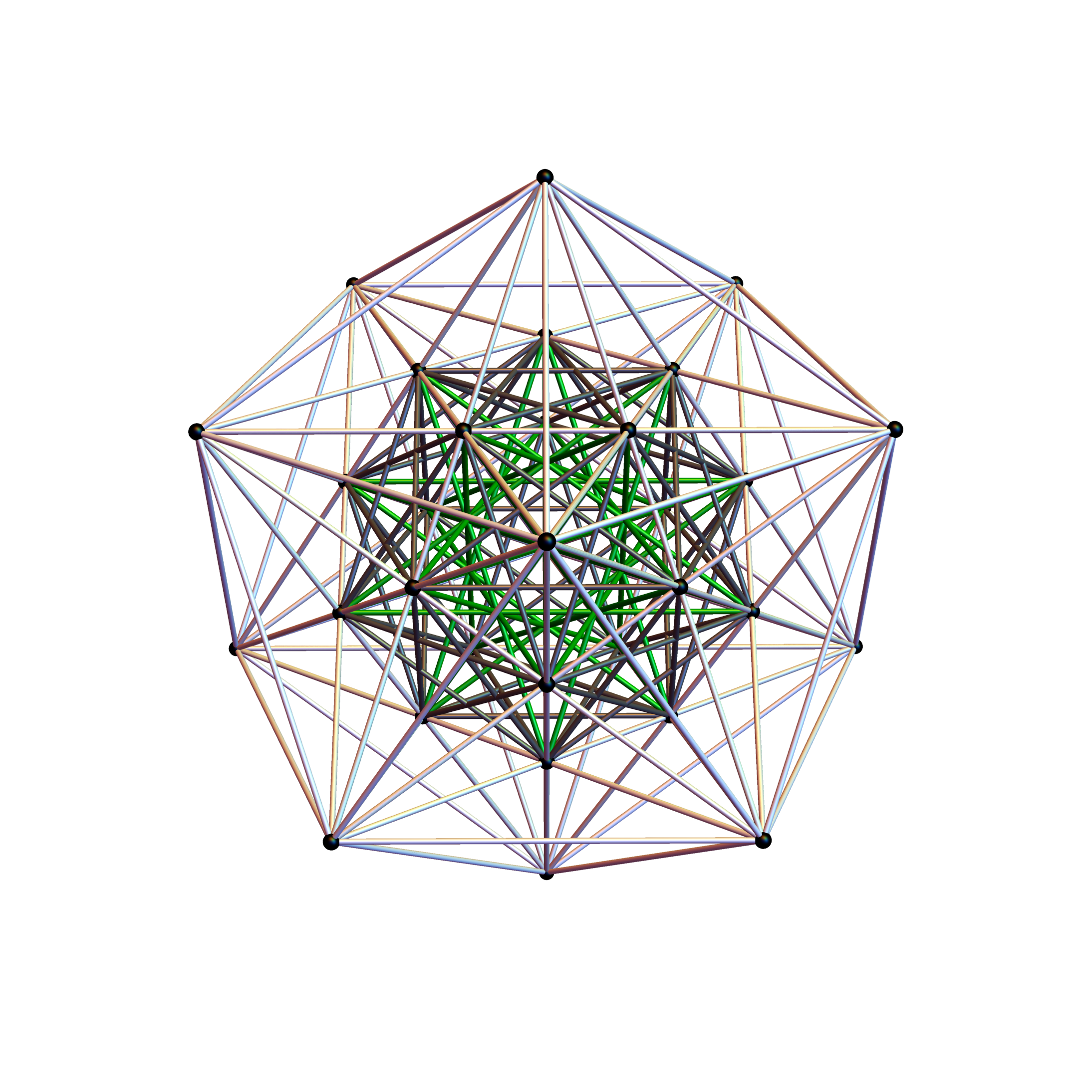# Rhombic Triacontahedron Animations

Best Viewed in HD

3D Stereoscopic

Red-Cyan Stereoscopic

BTW – if you find this information useful, or provide any portion of it to others, PLEASE make sure you cite this post. If you feel a blog post citation would not be an acceptable form for academic research papers, I would be glad to clean it up and put it into LaTex format in order to provide it to arXiv (with your academic sponsorship) or Vixra. Just send me a note at:  jgmoxness@theoryofeverthing.org.

# More Symmetries of E8 folding, including 5-Cube and 4-Cube (Tesseract)

The same 3 (projection basis vectors that produce the H3 Icosadodecahedron from D6 and the Rhombic Triacontahedron from the 6-Cube (or 2 sets from the 128 1/2 Integer BC8 vertices of E8) form lower dimensional objects within E8.

For more information on the above symmetries, see this post.

The 3D object identification has been confirmed by …

32 vertex 5-Cube with 80 5D Norm’d Unit Length Edges
Projection Basis Vectors {x,y,z}: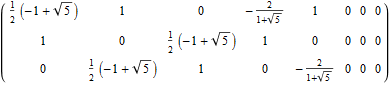3D Rhombic 20-Hedron Outer Hull of 22 Vertices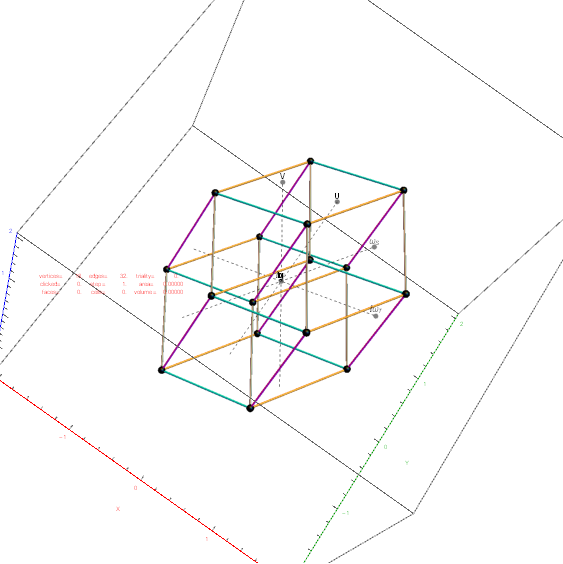The edge coloring on these projections are defined by which of the 6 dimensional axis the edge aligns with.

10 Interrior Vertices with 10 EdgesAll Vertices in 3D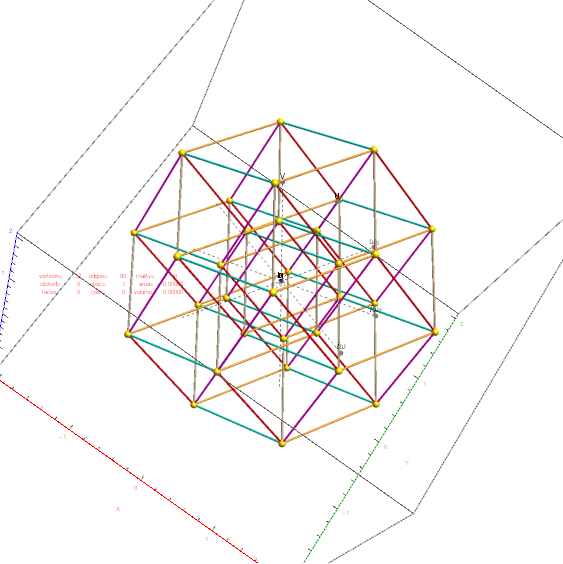16 vertex 4-Cube with 32 4D Norm’d Unit Length Edges
Projection Basis Vectors {x,y,z}: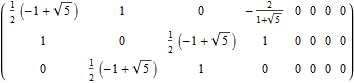3D Rhombic Dodecahedron with 2 Interior Vertices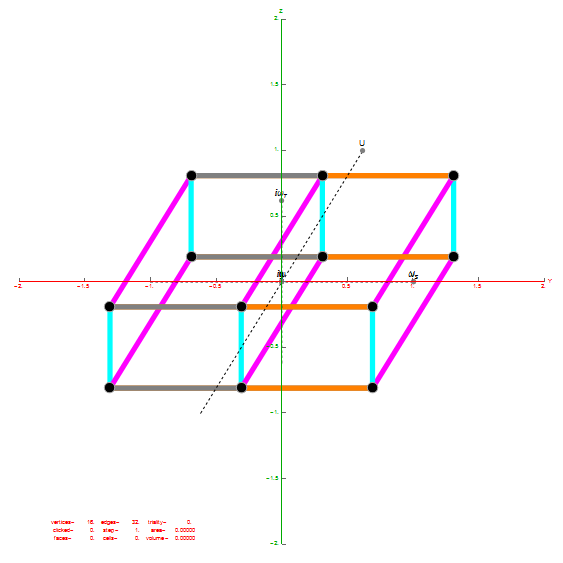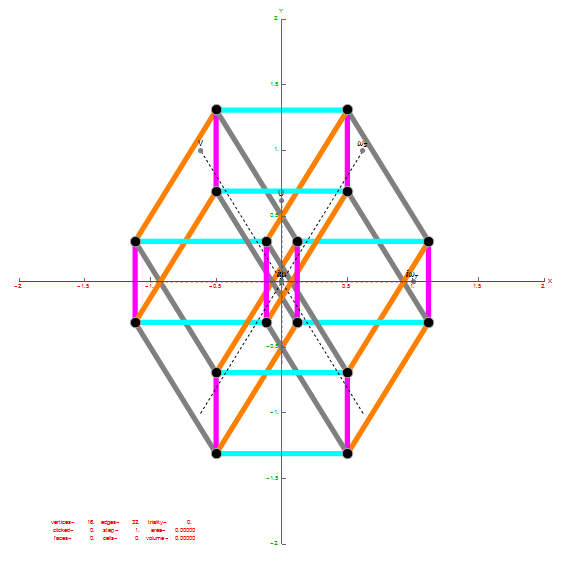BTW – if you find this information useful, or provide any portion of it to others, PLEASE make sure you cite this post. If you feel a blog post citation would not be an acceptable form for academic research papers, I would be glad to clean it up and put it into LaTex format in order to provide it to arXiv (with your academic sponsorship) or Vixra. Just send me a note at:  jgmoxness@theoryofeverthing.org.

# The Rhombic Triacontahedron and E8 to H4+H4Φ folding

I was asked to clarify a particular projection of the Rhombic Triacontahedron from the 6-Cube subgroup of E8, which had I created for WikiPedia (WP) back in 2011 based on an E8 to H4+H4Φ rotation matrix I discovered in 2010.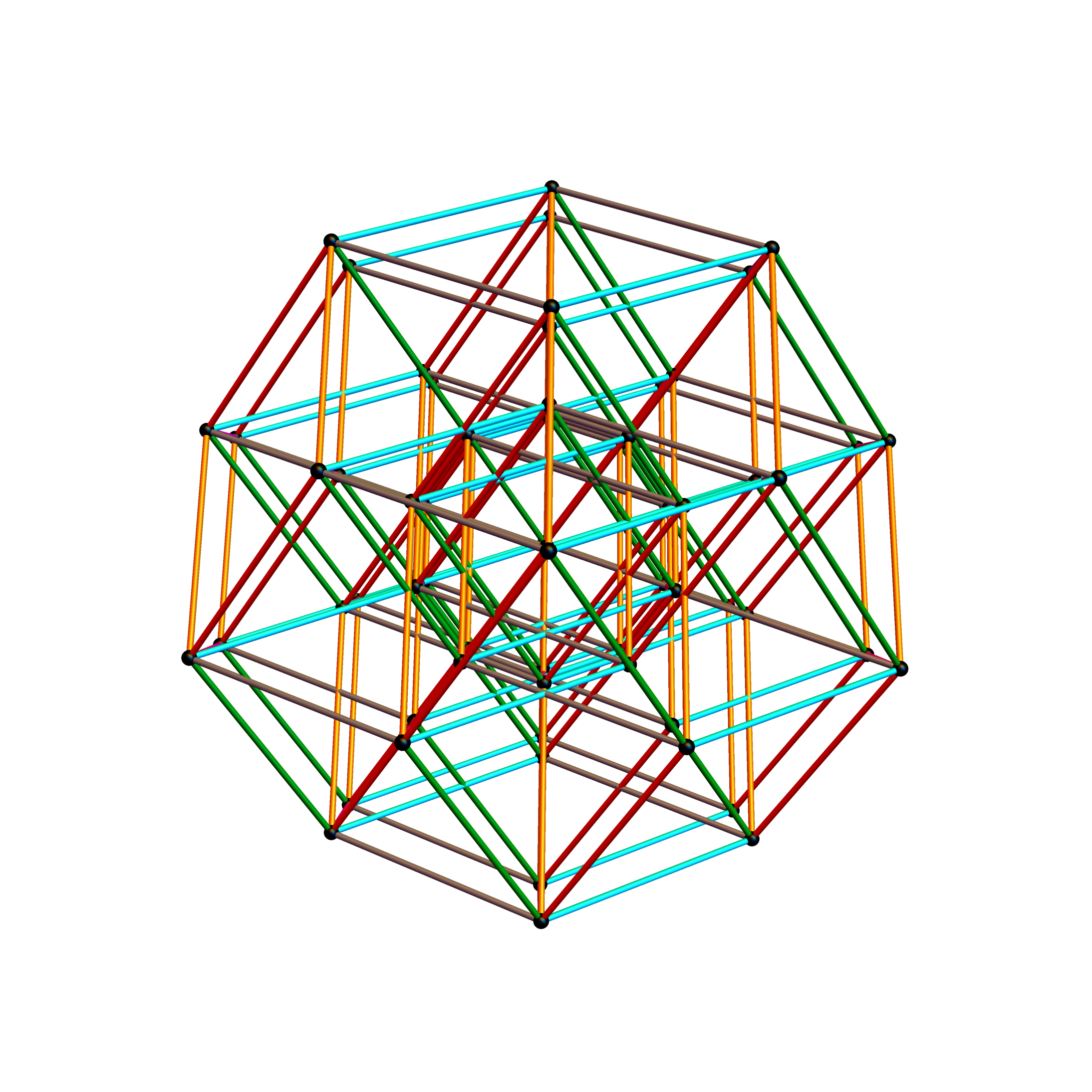The edge coloring on the projection above is defined by which of the 6 dimensional axis the edge aligns with.

BTW – if you find this information useful, or provide any portion of it to others, PLEASE make sure you cite this post. If you feel a blog post citation would not be an acceptable form for academic research papers, I would be glad to clean it up and put it into LaTex format in order to provide it to arXiv (with your academic sponsorship) or Vixra. Just send me a note at:  jgmoxness@theoryofeverthing.org.

The following projection (basis) vectors take the 6-Cube as a subset of E8 and projects it into 3D using the following basis vectors: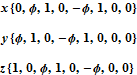Notice the last two dimensions in each vector are 0, which effectively takes the 128 8D 1/2 integer vertices of the E8 BC8 DemiCube into the 6D 6-Cube.

For the following data analysis, it is useful to reference the full list of E8 and H4+H4Φ data at the end of my blog post here.

#### The clarification desired was due to a conjecture that since E8 folds to H4+H4Φ (600 cells) and D6 folds to H3+H3Φ, that the 6-Cube should fold to an outer and inner rhombic triacontahedron scaled by Φ.

Below is a visualization of D6 folding into 2 concentric H3 icosadodecahedrons at a ratio of Φ using the same projection as described above. The edging displays the  480 Norm’d 6D Sqrt length edges, clearly showing the outer and inner H3 polytopes.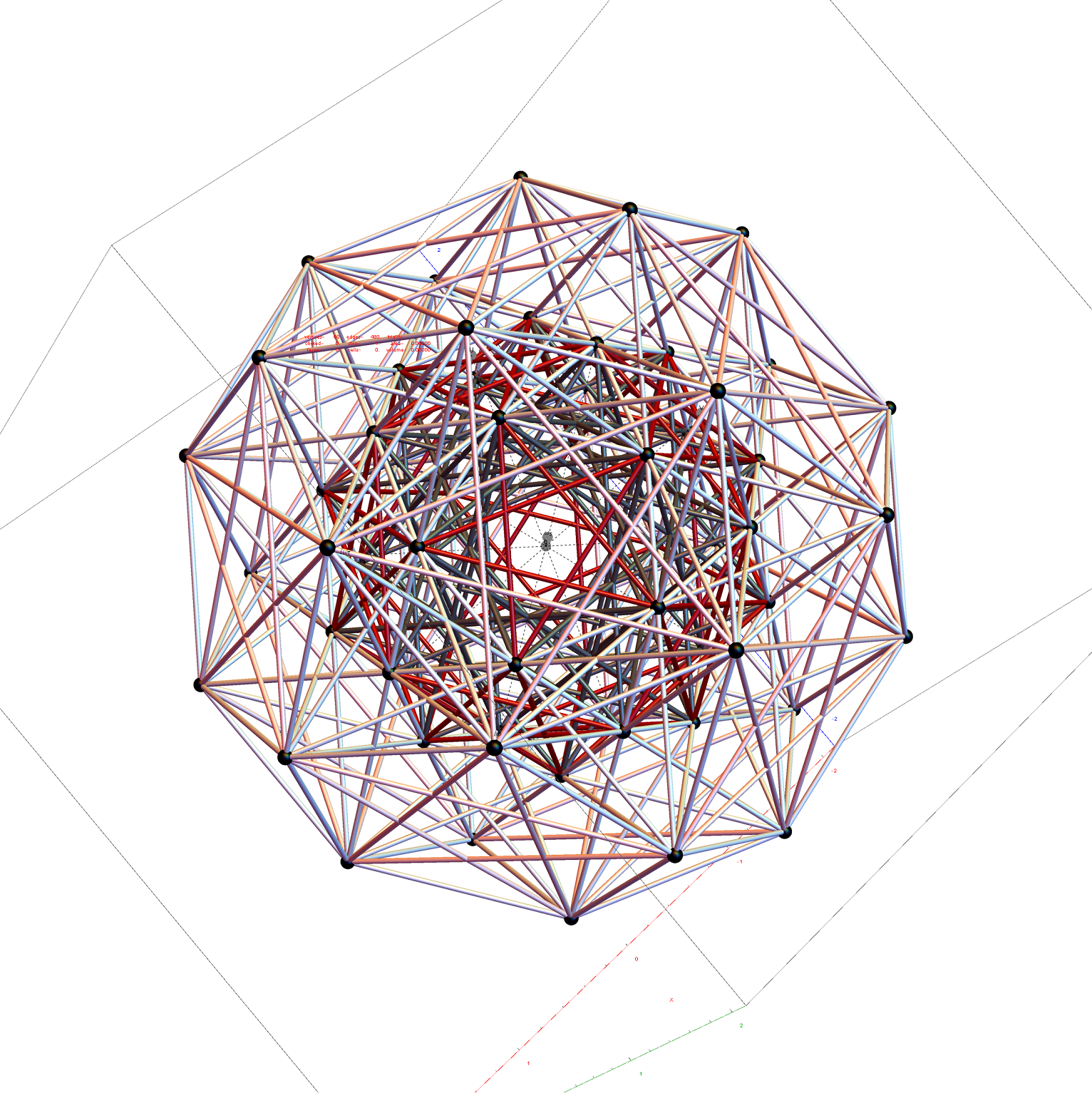Shown with exterior faces on edges (not a pure convex hull algorithm)…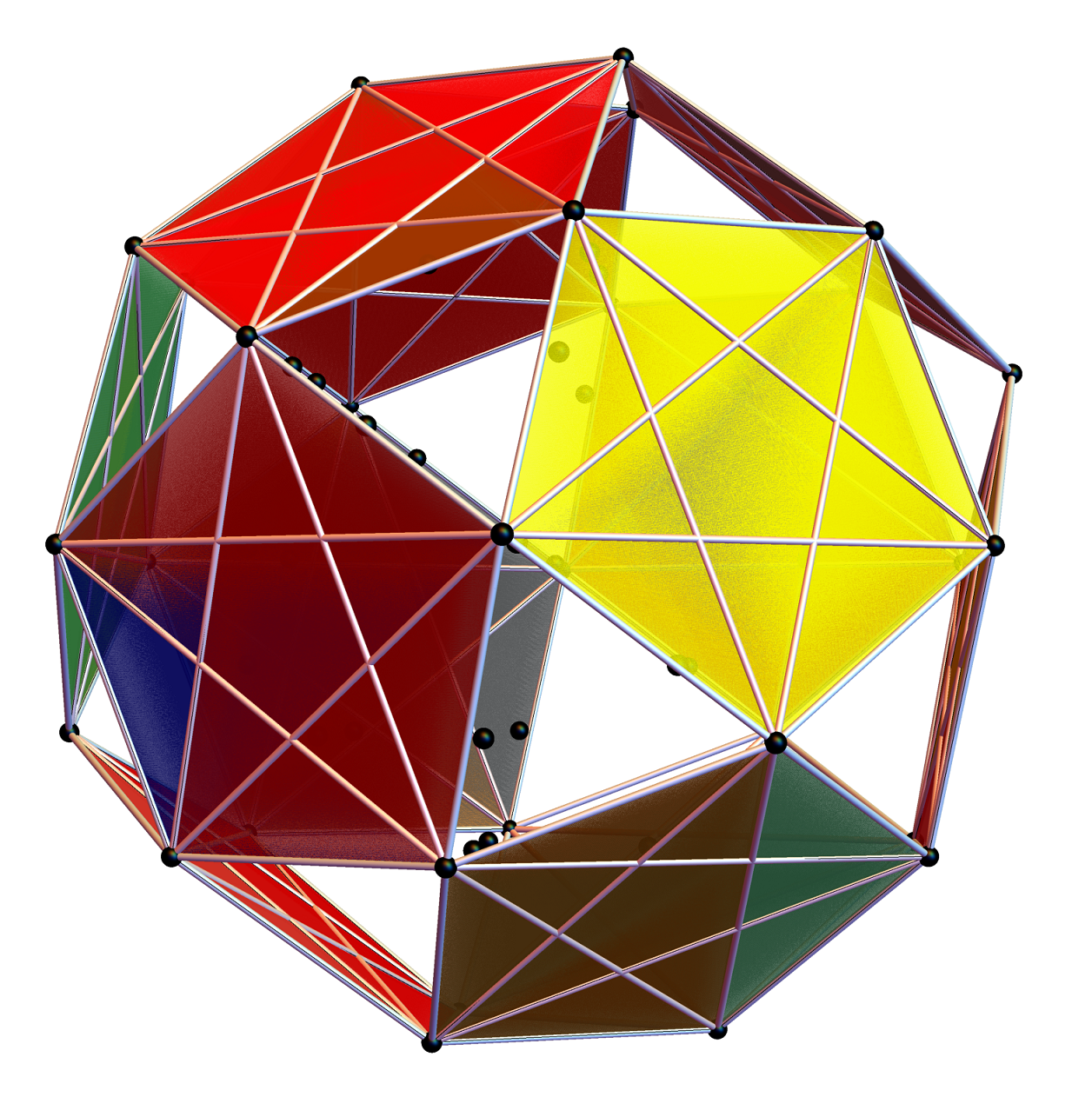The data below shows two sets of 30 vertices (outer on the left and inner on the right). They are sorted by the 3D Norm’d length of the vertex ray from {0,0,0}, followed by the vertex number in the E8 to H4+H4Φ list at the end of the post referenced above, along with the 6D E8 vertex that is a member of the D6 set, and the {x,y,z} projected vertex positionThe last line of the data set shows that the first set of outer 30 projected vertex locations on the left is numerically equal to the second inner set after multiplication by Φ.#### I did the same for the Rhombic Triacontahedron projected out of the 6-Cube.

I separated the outer and inner vertices used to make the WP projection at the top of the post. There are 64 vertices and 192 6D unit length edges forming pentagonal symmetry along specific axis (as well as hexagonal symmetries on other axis). It is clear that scaling the inner 32 vertex locations by Φ is not going to give the outer 32 vertex positions. Yet there is a similarity- they both have 12 vertices of one 3D Norm’d length and 20 of another.

Outer 32 vertices and 60 unit length Norm’d 6D edges.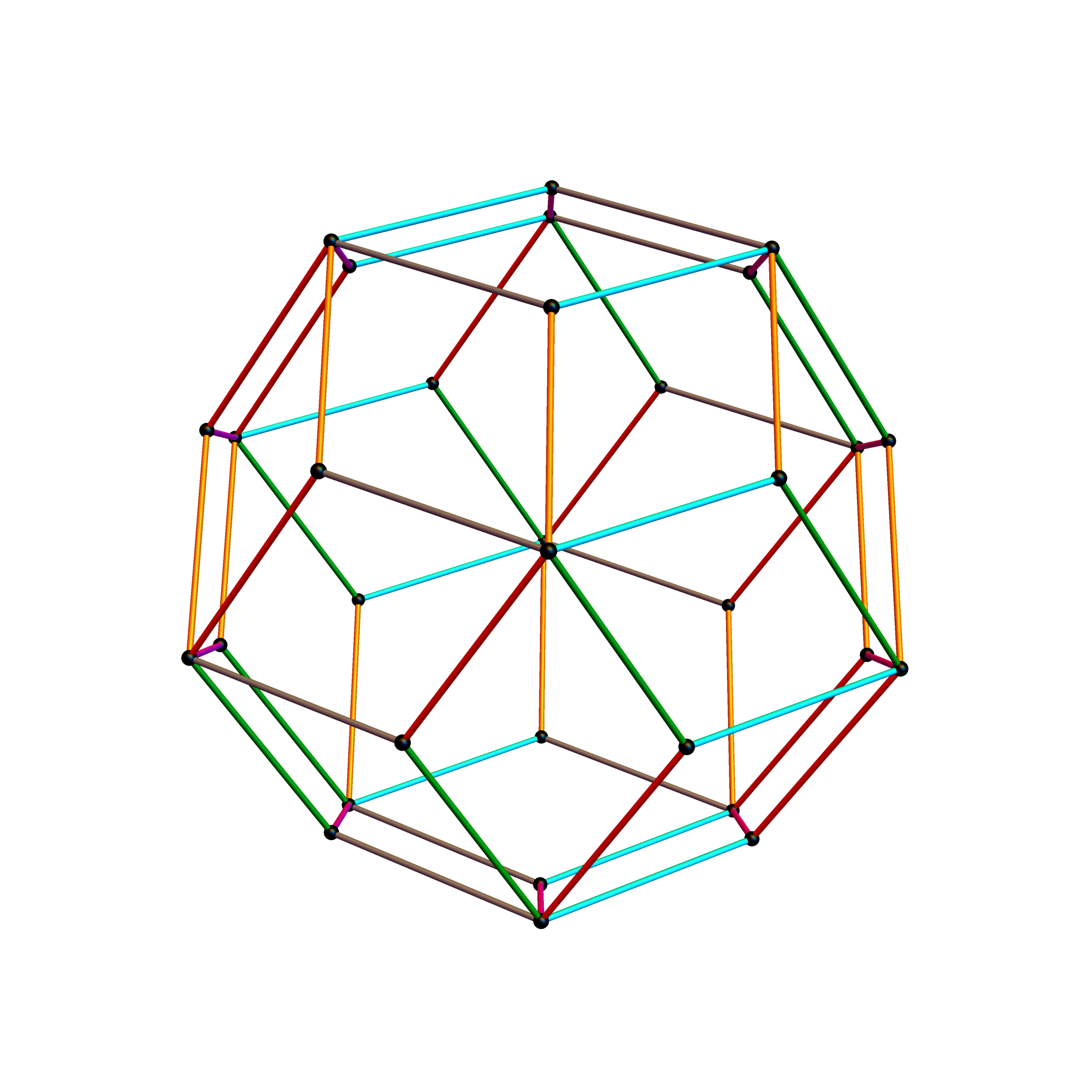The edge coloring on the projection above is defined by which of the 6 dimensional axis the edge aligns with.

Inner 32 vertices and 60 unit length Norm’d 6D edges.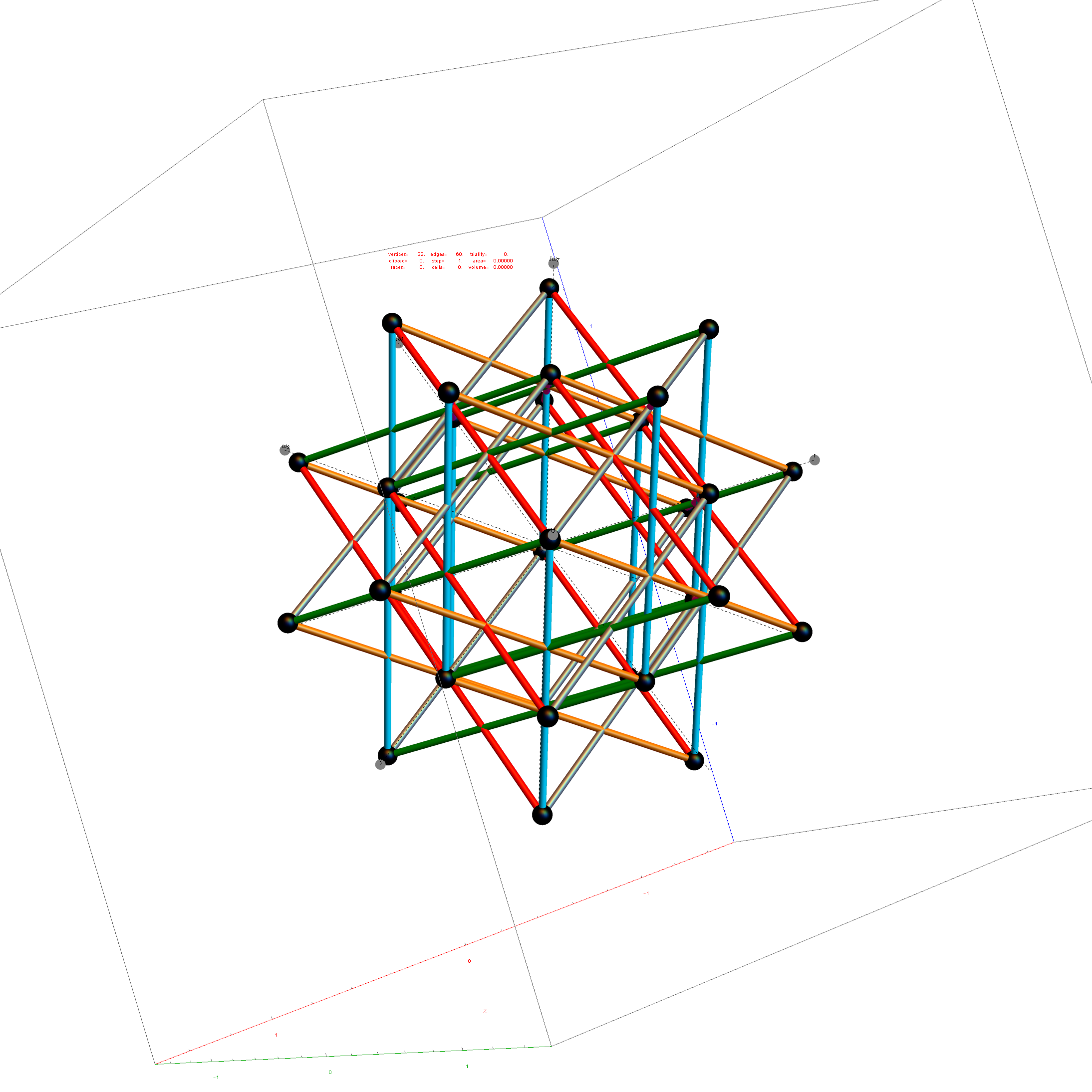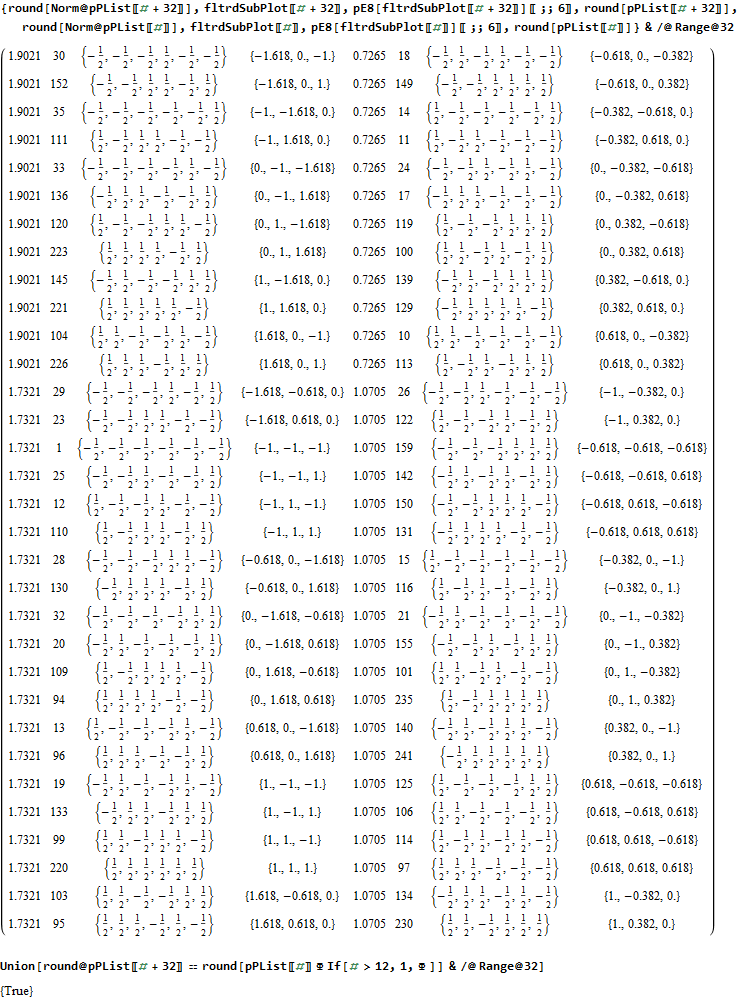The last line of the data set above shows that the outer data (left) is the same as the inner data set multiplied by a factor of Φ when the first 12 (shorter 3D Norm’d)  inner vertices (right) are multiplied by an additional factor of Φ.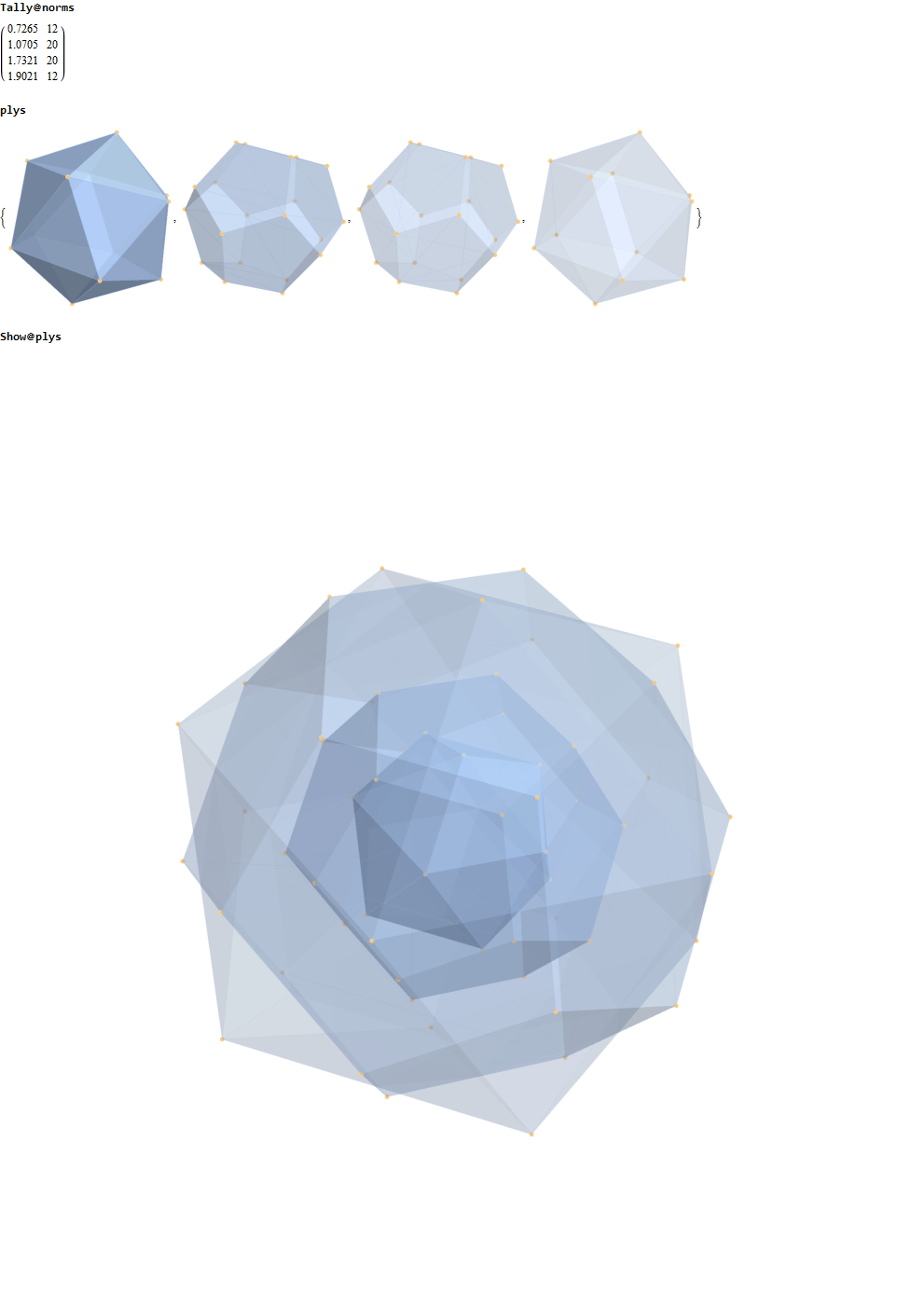#### This explains the lack of isomorphism, but why is it not like the D6 to H3+H3Φ and E8 to H4+H4Φ in the symmetry of Φ scaling?

The best explanation I can give involves the pattern of left vs. right 3 digits in the 6-Cube E8 vertices. This is due to the left right symmetry of the basis vectors applied to binary pattern of half integer vertices in the 6-Cube.

All vertices have a consistent pattern that differentiates the 20 longer Norm’d vertices from the 12 shorter ones. Specifically, the shorter 12 that have the inner ones needing the added factor of Φ always show that:

• the right inner vertices all have an even number in 6 +/- elements but not always evenly split across the left and right
• the left outer vertices all have an odd number of in 6 +/- elements  always unevenly split across the left and right

Whereas, the longer 20 that match the Φ scaling reverse the pattern where:

• the right inner vertices all have an odd number in 6 of +/- elements  always unevenly split across the left and right
• the left outer vertices all have an even number in 6 of +/- elements but not always evenly split across the left and right

Another related fact in the folding pattern of the 6-Cube emerges when we note that since E8 contains 128 1/2 integer BC8 (the 8 Demi-Cube). In addition, we note that the 6D complement of BC8 and the 6-Cube is the same set of vertices. This means we get the same projection using all 128 vertices of the 6D trimmed BC8 as we do with the 64 of the 6-Cube, except now there are 768 6D Norm’d unit length edges rather than 192. Interestingly, that means that similar to E8 containing simultaneous copies of 4 600-Cells (a left and right pair scaled at Φ), E8 contains 4 simultaneous copies of the rhombic triacontahedron after adding the Φ scaling on the two sets of 12 inner BC8 vertices).

#### Wow – that is nice! Remember, you heard it here first!

If we try this idea with the complement of the integer elements of E8, namely the 112  6D trimmed D8 vertices complemented with 60 D6 vertices, the 6D trimmed 52 vertex complement contains 4 identical copies of the excluded 16 generator vertices of E8 {+/-1,0,0,0,0,0,0,0} permutations (plus 4 {0,0,0,0,0,0,0,0} vertices).

As you can see, the folding of the integer elements of E8 do not fold the same as the 1/2 integer elements, and in retrospect, we probably should not expect them to.

# E8 folding to 4D H4+H4Φ Visualization Data Analysis

Below is an animated .GIF (3 seconds per frame) in 2D of E8 in Petrie projection with numbered vertices. It cycles between the full E8 (Gosset polytope), to the 8 16-Cell (Cross polytope) vertices, to the 16 8-Cell (Tesseract) vertices. It then cycles through the 5 H4+H4Φ 600-Cell pairs of 24-Cells (main 24-Cell and 4 Snub 24-Cells rotated around the Petrie projection).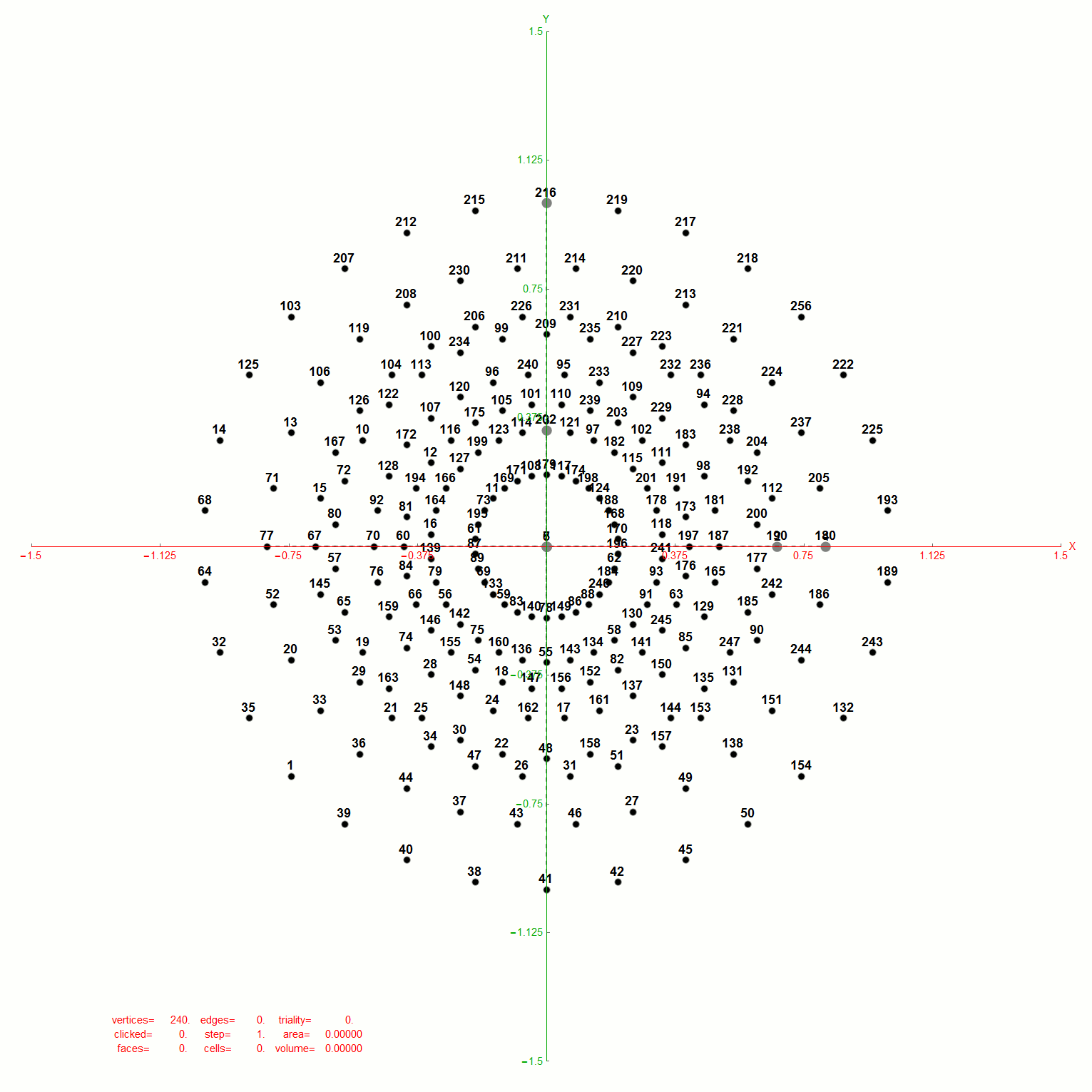Notice that 4 of the 8 16-Cell vertices are co-located on the axes with the basis vectors of the 2D projection. The other 4 basis vectors (on the right half of the 8D basis) are located at the origin {0,0}.

BTW – if you find this information useful, or provide any portion of it to others, PLEASE make sure you cite this post. If you feel a blog post citation would not be an acceptable form for academic research papers, I would be glad to clean it up and put it into LaTex format in order to provide it to arXiv (with your academic sponsorship) or Vixra. Just send me a note at:  jgmoxness@theoryofeverthing.org.

The Split Real Even (SRE) E8 vertices are numbered in order of their canonical sort position in the Pascal Triangle binary order. See the large .PNG graphic at the bottom of this post for the full list of binary, E8 and folded H4+H4Φ in symbolic and numeric values. The connection between E8 and the binary (binomial expansion) of the Pascal Triangle (Clifford Algebra) is visualized here. It is interesting to note that the binary and E8 vertex values of 1-128 are mirrored by the 256-129 (note: reverse order, with 0<->1 for binary or negation for E8).

The H4 numeric and symbolic information is obtained through E8 vertex dot product with the 8×8 rotation matrix and is multiplied by 2 for clarity. H4 Folding is obtained by only using the top or bottom 4 rows of that matrix I discovered in 2010 (below):Notice the Quaternion to Octonion Cayley-Dickson like doubling procedure pattern in the 4 quadrants of this matrix. It is this pattern that is responsible for maintaining the 4 sets of 600-Cell H4 vertices when rotating from E8 vertices.

Below is the same type of animated .GIF from above, except it is projected into 3D by adding a 3rd basis vector for the z axis. These are shown without vertex numbers for clarity. It starts with E8 with 6720 8D Norm=Sqrt edges (or equivalently E8 rotated to H4+H4Φ edges with 3360 4D Norm=1 & 3360 4D Norm=Φ edges). It cycles through to the H4Φ 600-Cell, H4 600-Cell, and then to the 24-Cell without edges. Finally on to the 8-Cell and 5 pairs of 24-Cells with edges. Again, the right 4D half of the 8D projection basis vectors are located at {0,0,0}. Of the other 4 basis vectors, 2 are on {x,y,z} axes.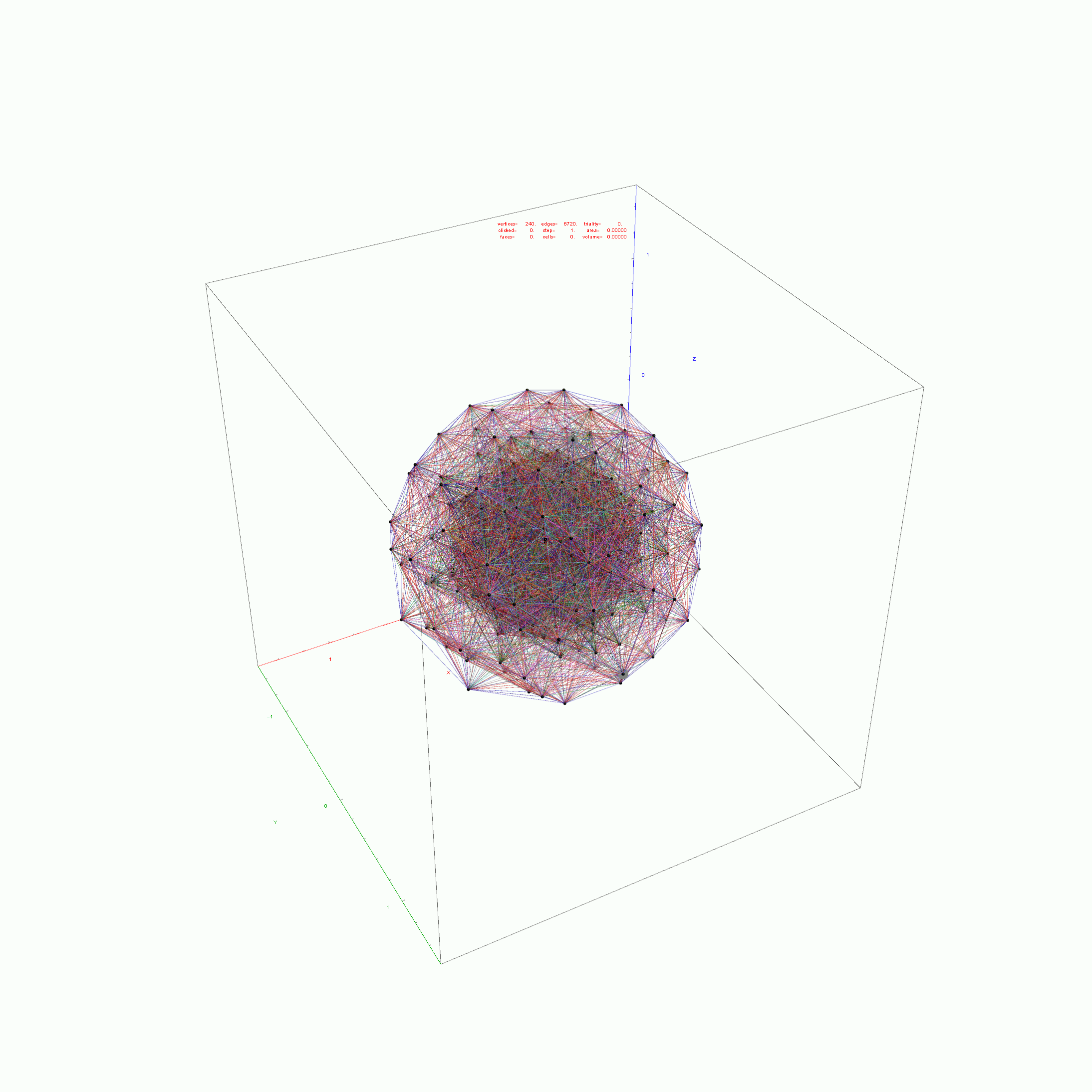The projections of E8 rotated to H4+H4Φ in this post are not a pure ortho-normal 600-Cell projection, in that it is intended to show the Petrie projection on one face {x,y}. It does show the ortho-normal 600-Cell structure on another face {y,z} (albeit rotated off axis), and something in-between these on the {x,z} face. The full ortho-normal 600-Cell can easily be projected to full 3D symmetry on all 6 faces by using an ortho-normal basis. The 3 projection basis vectors used here in this post are as follows: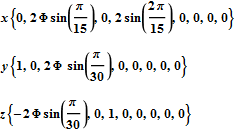Below is the very long .PNG graphic listing the left 4D and right 4D vertex information for E8 (Pascal Triangle Binary and Split Real Even E8) and folded H4 and H4Φ in symbolic and numeric form. On the left side is the vertex number. Notice vertices 2-9 and 248-255 are missing. These are the 8 generator (and anti-generator) E8 vertices. The center of each row is a number that identifies the vertex where the left and right 4D elements switch sides to right and left.

The green data in the left and right columns indicate a 4D H4 vertex, while black in those columns indicate H4Φ 4D vertex. As you can see, there are actually 4 H4 600-Cell constructs in this E8 rotation, with 2 on the left and 2 on the right.

#### Wow – that is nice! Remember, you heard it here first!

The red text in the center column indicates vertices of the H4Φ 24-Cell. Notice, all the red vertices don’t switch left/right since they have symmetric 4D halves. Interestingly, 1/2 of the 16 H4Φ 8-Cell vertices are made up of 1/2 of the 16 8-bit Hamming (distance 4) binary codes. The other 1/2 of those Hamming codes are located in the 16 H4 8-Cell vertices, with anti-symmetric left/right halves (described in the next paragraph).

The Orange is the H4 24-Cell. While this 24-Cell does have a different vertex identified in the center for left/right switching, it does so within H4. Another interesting observation, is that both the E8 and H4 left value is always the negative of the right. This means the center number that identifies the vertex where the left and right 4D elements switch sides is the same as the 1-128 mirror element in the 256-129 set (and vice-versa).

The 2*8 (red and orange) 16-Cell vertices are all located in the integer E8 vertices (groups 4 and 6 of row 9 of the Pascal Triangle, specifically (1,8*,28,56,70,56,28,8*,1)). The 2*16 (red and orange) vertices of the 8-Cells are all located in the 1/2 integer E8 vertices groups (1,3,5,7,9) of row 9 of the Pascal Triangle, with all 16 of the H4 8-Cell vertices in group 5, and with the 16 red H4Φ 8-Cell vertices distributed as {1,4,6,4,1) in groups (1,3,5,7,9) respectively. Notice that this {1,4,6,4,1) distribution may relate to the Clifford Algebra Cl(8) primitive idempotent distributions in the 256 elements of Pascal Triangle row 9.

These red and orange center patterns of the 24-Cells is unlike the 2 sets of 96 Snub 24-Cell vertices, which have both H4 and H4Φ elements in each row as well as switching left/right values with a different and non-mirrored vertex number in the center of the center column.

More visualizations and 600 Cell analysis is also found in this post.

Please notice I have left in the final command of the Mathematica source code to give a hint as to the source structure of the data.